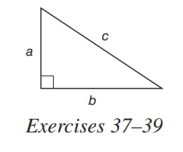Chapter A.5, Problem 37EElementary Geometry For College St...

7th Edition
Alexander + 2 others
ISBN: 9781337614085

Solutions

Chapter
SectionElementary Geometry For College St...

7th Edition
Alexander + 2 others
ISBN: 9781337614085
Textbook Problem

In Exercises 37-39, c 2 = a 2 + b 2 .In the right triangle, find c if a = 3     a n d     b = 4 .To determine

To find:

To find c if a=3andb=4.

Explanation

Consider the following equation,

c2=a2+b2.

The diagrammetic representation is given below,

Substitute the values of a=3andb=4 in the above equation to get the following,

c2=a2+b2c2=32+42c2

Still sussing out bartleby?

Check out a sample textbook solution.

See a sample solution

The Solution to Your Study Problems

Bartleby provides explanations to thousands of textbook problems written by our experts, many with advanced degrees!

Get Started

In Exercises 11-16, find the slope of the line that passes through each pair of points. 13. (2, 3) and (4, 8)

Applied Calculus for the Managerial, Life, and Social Sciences: A Brief Approach

If g(x) = 3 + x + ex, find g1(4).

Single Variable Calculus: Early Transcendentals

π does not exist

Study Guide for Stewart's Single Variable Calculus: Early Transcendentals, 8th